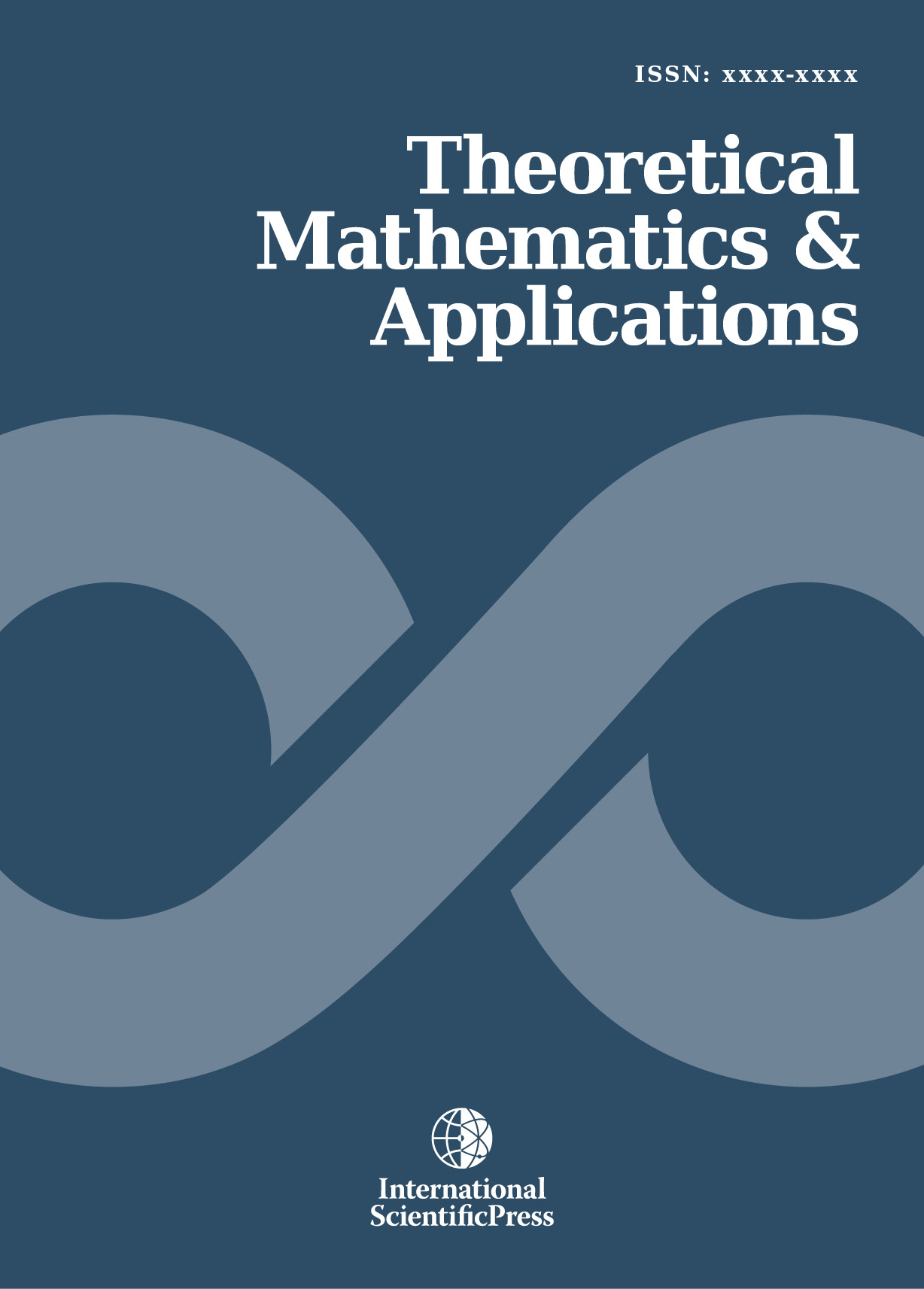# Theoretical Mathematics & Applications

#### The Approximate Method for Solving Poincare Problem of Nonlinear Elliptic Equations of Second Order

•[ Download ]
• Times downloaded: 1071
• Abstract

This article deals with the approximate method used to solve the Poincar´e boundary value problem for nonlinear elliptic equations of second order in unbounded multiply connected domains. This type of boundary value problems are known to have applications in many fields such as mechanics and physics. We first present a formulation of the boundary value problem and the corresponding modified well-posedness. Then we obtain the representation theorem and a priori estimates of solutions for the modified problem. Finally by the estimates of solutions and the continuity method, we obtain the solvability results and error estimates of approximate solutions of the modified problem for the nonlinear elliptic equations of second order.ISSN: 1792-9687 (Print)
1792-9709 (Online)